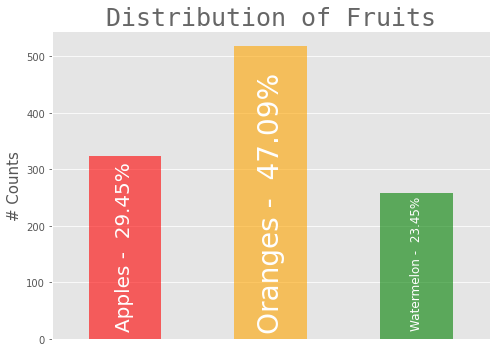# How to create a variable fontsize for bar plot annotations

How to choose the font size for text annotations inside the bars of the bar graph with the condition:

• Text will completely cover the rectangular bar area.

Please go through the diagram and code for better clarity about the problem.So, the requirement is only : font size should be relative to bars in the bar graphs

Code

```import pandas as pd
import numpy as np
import matplotlib.pyplot as plt
import matplotlib as mpl

# Plot styles
mpl.style.use("ggplot")

# data
fruits = pd.Series(index = ["Apples", "Oranges", "Watermelon"], data = [324,518, 258])

# Bar graph for Fruits

# figure
plt.figure(figsize = (7,5))

# bar graph
fruits.plot(kind = "bar", color = ["red", "orange", "green"], alpha = 0.6, width = 0.5, )

# percentage of each fruit type
categories = list(fruits.index)
categories_percent = [100*(value/fruits.sum()) for value in fruits ]

# categories annotations coordinates
ax = plt.gca() # get current axes
rects = ax.patches # rectangles axes of bars in the graph

# annotations
for i in range(len(categories)):
plt.annotate(f"{categories[i]} - {categories_percent[i] : 0.2f}%",
xy = (rects[i].get_x() + rects[i].get_width()/2,
rects[i].get_y() + (ax.get_yticks() - ax.get_yticks())*.2),
fontsize = [20,28,12][i], # Chosen by hit and trial for adjustment
color = "white",
ha = "center",
rotation = 90,
)

plt.ylabel("# Counts", fontsize = 15,)
plt.title("Distribution of Fruits", fontsize = 25, fontname = "Monospace", alpha = .6)
plt.xticks([])
plt.tight_layout(rect=[0, 0, 1, 1])
plt.show()
```

How to deal with this line of code `fontsize = [20,28,12][i], # Chosen by hit and trial for adjustment` to adjust the font size dynamically with respect to bar area?

• Updating the existing annotation with an adjustable `fontsize`
• From a logical perspective figure sizes’ `y` acts as a scaling factor for height.
• Think `.get_height` as a relative height of the figure.
• The actual height is the y scaling factor multiplied with `.get_height`.
• About including breadth, we can include relative breadth which is just `.get_width` (not `get_width*x`), however it would just act as a constant, since it’s relative width.
• We can’t include actual width because the font would adjusted unproportionally for y axis.
```x,y=15,15
plt.figure(figsize = (x,y))

for i in range(len(categories)):
txt="{} - {: 0.2f} %".format(categories[i],categories_percent[i])
plt.annotate(txt,
xy = (rects[i].get_x() + rects[i].get_width()/2,
rects[i].get_y() + (ax.get_yticks() - ax.get_yticks())*.2),
fontsize = (rects[i].get_height())*y*.2/len(txt), # Chosen by hit and trial for adjustment
color = "white",
ha = "center",
rotation = 90,
)
```
• The entire code can be written more cleanly as follows
```# data
fruits = pd.Series(index = ["Apples", "Oranges", "Watermelon"], data=[324,518, 258])

# calculate percent
per = fruits.div(fruits.sum()).mul(100).round(2)

# bar graph
y = 5
ax = fruits.plot(kind="bar", color=["red", "orange", "green"], alpha=0.6, width=0.5, figsize=(7, y), rot=0)

labels = [f'{fruit} - {per[fruit]}%' for fruit in fruits.index]

# annotations:
for label, p in zip(labels, ax.patches):
left, bottom, width, height = p.get_bbox().bounds
fs = height * y * 0.18 / len(label)
ax.annotate(label, xy=(left+width/2, bottom+height/2), ha='center', va='center', rotation=90, fontsize=fs)

plt.ylabel("# Counts", fontsize=15,)
plt.title("Distribution of Fruits", fontsize=25, fontname="Monospace", alpha=.6)
plt.xticks([])
plt.tight_layout(rect=[0, 0, 1, 1])
plt.show()
```

For figsize=(15,15):For figsize=(8,8):For figsize=(7,5):